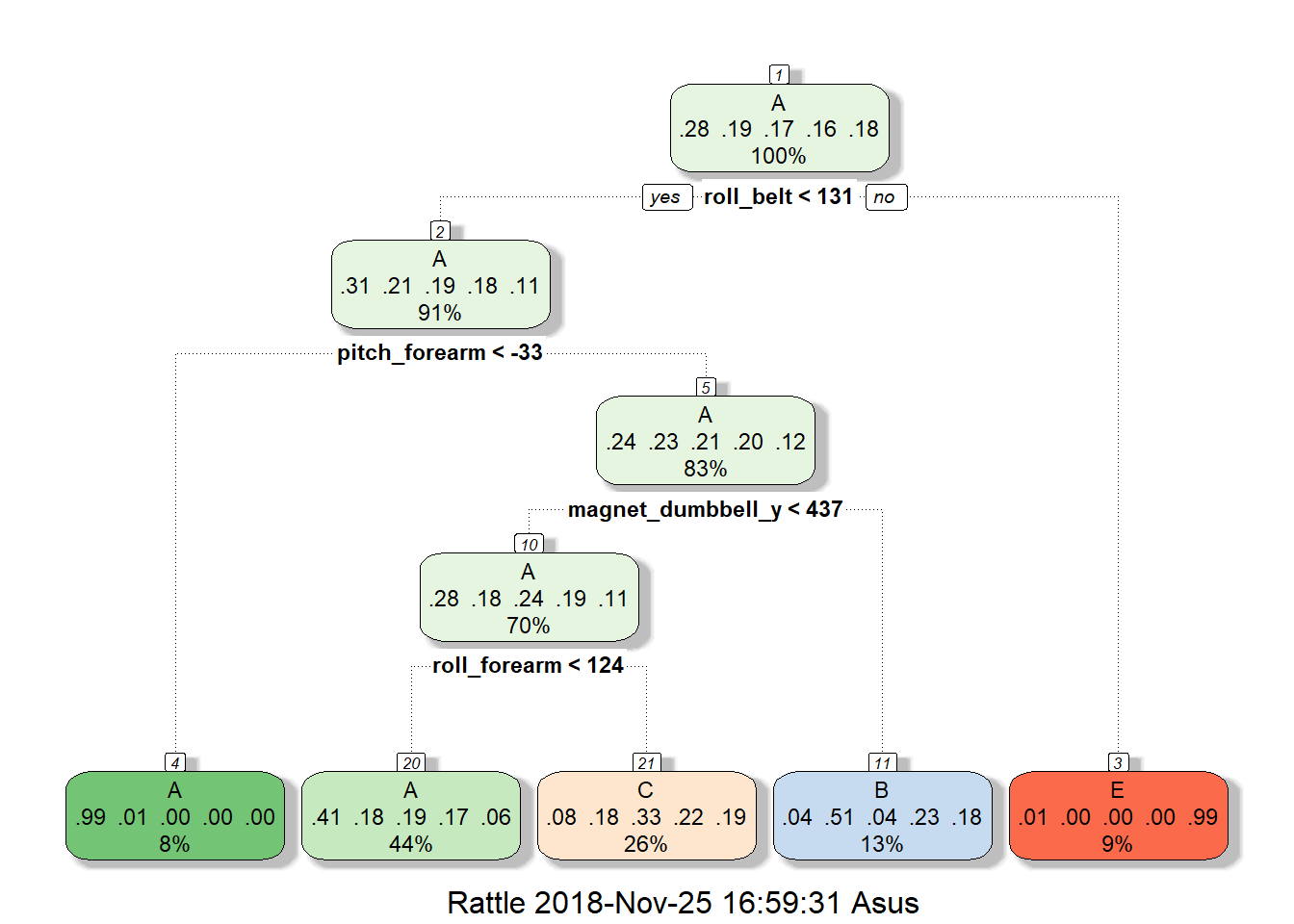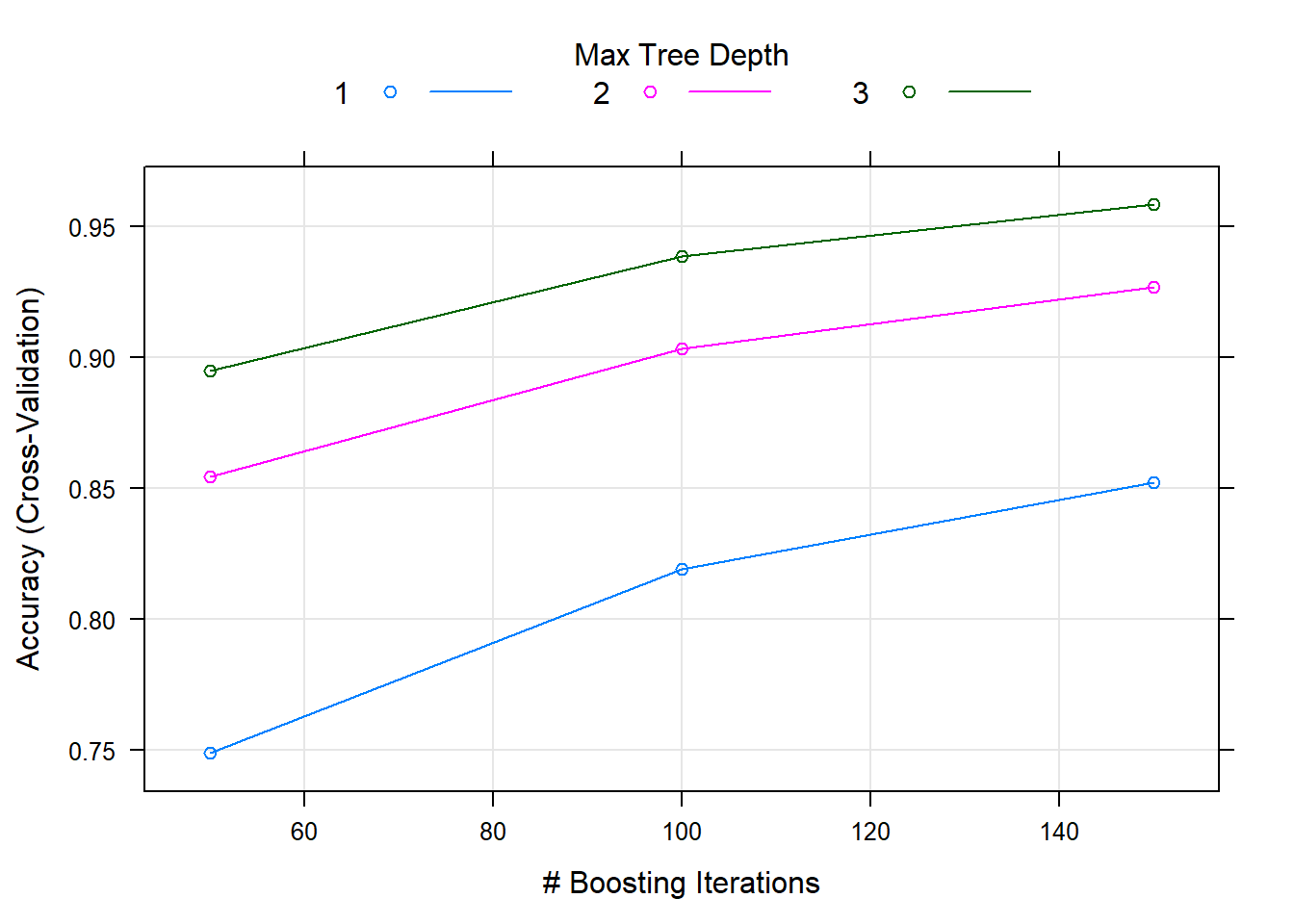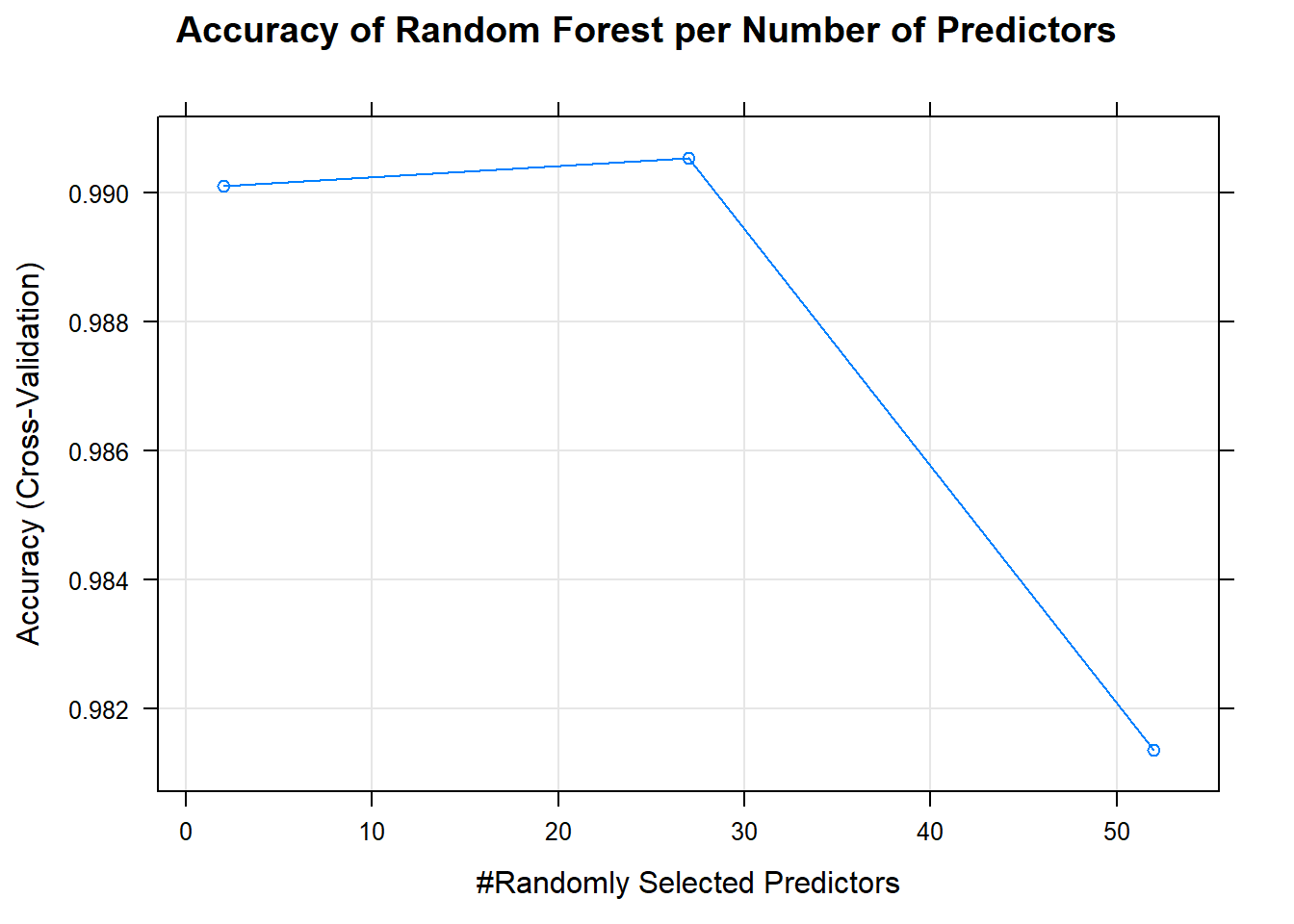## Overview

This is my report for the project on practical machine learning for the coursera course offered by Johns Hopkins University.

### Loading the required packages and the dataset for both training and testing

library(caret)
## Loading required package: lattice
## Loading required package: ggplot2
library(rattle)
## Rattle: A free graphical interface for data science with R.
## Version 5.2.0 Copyright (c) 2006-2018 Togaware Pty Ltd.
## Type 'rattle()' to shake, rattle, and roll your data.
training <- read.csv("pml-training.csv")
testing <- read.csv("pml-testing.csv")

## Viewing the datasets to determine the structure of variables

##Results are hidden to save space
str(training)
str(testing)
##training data has 19622 obs. of  160 variables
##testing data has 20 obs. of  160 variables
##It can also be observed that there were many columns that have NAs so it is time to clean the dataset.

## Cleaning the Dataset for both training and testing

##Removing unwanted columns; those with 90% NAs
RemoveNAtest <- which(colSums(is.na(training) |training == "")>0.9*dim(training))
RemoveNAtrain <- which(colSums(is.na(testing) |testing == "")>0.9*dim(testing))
Ctrain <- training[, -RemoveNAtrain]
Ctest <- testing[, -RemoveNAtest]

##Removing unwanted columns; those pertaining to user profile which is not relevant to prediction
Ctrain <- Ctrain[,-c(1:7)]
Ctest <- Ctest[,-c(1:7)]
##Both training and testing datasets now have 53 variables

## Data Splitting to remove out-of-sample errors

##Ctrain is split into 70% for training and 30% for validation
set.seed(7788)
inBuild <- createDataPartition(Ctrain$classe, p = 0.7, list = 0) Btrain <- Ctrain[inBuild,] Bval <- Ctrain[-inBuild,] ## Cross Validation ##Setting up the cross validation with 5-fold cross validation ##This improves model efficiency and limits overfitting Ctrl <- trainControl(method = "cv", number = 5) ## Prediction Models This report considers three models for prediction which includes classification tree using “rpart”, random forest using “rf” and gradient boosting with “gbm”. ### Classfication Tree modCT <- train(classe~., data = Btrain, method = "rpart", trControl = Ctrl) ##Create a nice plot fancyRpartPlot(modCT$finalModel)##Predict outcomes using the validation dataset
predCT <- predict(modCT, Bval)
##Check using Confusion Matrix
cmCT <- confusionMatrix(predCT, Bval$classe) aCT <- cmCT$overall['Accuracy']
print(aCT)
##  Accuracy
## 0.4907392
##Low accuracy at 49.07% thus poor prediction of the outcome "classe"

modGBM <- train(classe~., data = Btrain, method = "gbm", trControl = Ctrl, verbose = 0)
##Create a nice plot
plot(modGBM)##Predict outcomes using the validation dataset
predGBM <- predict(modGBM, Bval)
##Check using Confusion Matrix
cmGBM <- confusionMatrix(predGBM, Bval$classe) aGBM <- cmGBM$overall['Accuracy']
print(aGBM)
##  Accuracy
## 0.9653356
##We see a high accuracy at 96.53% but before we decide let us check the random forest

### Random Forest

modRF <- train(classe~., data = Btrain, method = "rf", trControl = Ctrl)
##Create a nice plot
plot(modRF, main = "Accuracy of Random Forest per Number of Predictors")##Predict outcomes using the validation dataset
predRF <- predict(modRF, Bval)
##Check using Confusion Matrix
cmRF <- confusionMatrix(predRF, Bval$classe) aRF <- cmRF$overall['Accuracy']
print(aRF)
## Accuracy
## 0.993373
##Comparing the previous Accuracy we have computed, it looks like random
##forest has the highest accuracy at 99.34%. As such, we will be using
##random forest for prediction on the test dataset (Ctest).

## Prediction on Test dataset using Random Forest

Testpred <- predict(modRF, Ctest)
print(Testpred)
##   B A B A A E D B A A B C B A E E A B B B
## Levels: A B C D E

## Conclusion

Three prediction models were considered to predict classe in the test dataset. These models include Classification Tree, Generalized Boosting Method and Random Forest. A 5-fold cross validation method was considered for efficiency purposes (faster processing) and to limit overfitting. Going over the specified models, the following accuracy were observed as 49.07% for Classification Tree, 96.53% for Generalized Boosting Method and 99.34% for Random Forest. This informs us that random forest has the lowest out-of-sample error at .0067 followed by Generalized Boosting Method at 0.035 and 0.509 for Classification Tree. With the following findings, it is wise to decide on the use of Random Forest to predict classe in the test data set given its high accuracy and low out-of-sample error.